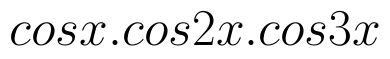Select Page

# CBSE Maths 12 Science Matrices MCQ Solutions in English

CBSE Maths 12 Science Matrices MCQ Solutions in English to enable students to get Solutions in a narrative video format for the specific question.

Expert Teacher provides CBSE Maths 12 Science Matrices MCQ Solutions through Video Solutions in English language. This video solution will be useful for students to understand how to write an answer in exam in order to score more marks. This teacher uses a narrative style for a question from Matrices not only to explain the proper method of answering question, but deriving right answer too.

Please find the question below and view the Solution in a narrative video format.

Question:

Solution Video in English:

You can select video Solutions from other languages also. Please check Solutions in ( Hindi )

## Similar Questions from CBSE, 12th Science, Maths, Matrices

Question 1 : If A is a square matrix such that,then find the simplified value of:. (View Answer Video)

Question 2 : Given,, find the value of w. (View Answer Video)

Question 3 : If, write the value of"x". (View Answer Video)

Question 4 : Find the value of z from the equation:(View Answer Video)

Question 5 : If, find (x-y). (View Answer Video)

### Application of Derivatives

Question 1 : The total revenue in rupees received from the sale of x units of a product is given by. The marginal revenue, when x=15 is: (View Answer Video)

Question 2 : The normal to the curvepassing (1, 2) is: (View Answer Video)

Question 3 : The volume of a cube is increasing at the rate of 9 cubic centimetres per second. How fast is the surface area increasing when the length of an edge is 10 centimetres? (View Answer Video)

Question 4 : The maximum value ofis (View Answer Video)

Question 5 : Find the maximum profit that a company can make if the profit function is.(View Answer Video)

### Continuity and Differentiability

Question 1 :  Find the second order derivative of the function. (View Answer Video)

Question 2 : Findfor the function. (View Answer Video)

Question 3 :  Differentiate w.r.t.x the function. (View Answer Video)

Question 4 : Differentiatew.r.t.x. (View Answer Video)

Question 5 : Differentiate the function w.r.t.x. (View Answer Video)

### Linear Programming

Question 1 : The objective function is maximum or minimum, which lies on the boundary of the feasible region. (View Answer Video)